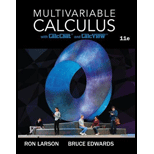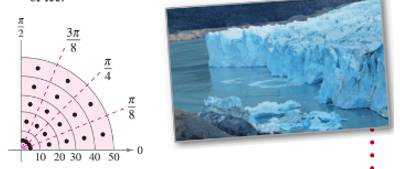# Glacier Horizontal cross sections of a piece of ice that broke from a glacier are in the shape of a quarter of a circle with a radius of approximately 50 feet. The base is divided into 20 subregions, as shown in the figure. At the center of each sub region, the height of the ice is measured, yielding the following points in cylindrical coordinates. ( 5 , π 1 6 , 7 ) , ( 15 , π 1 6 , 8 ) , ( 25 , π 1 6 , 10 ) , ( 35 , π 1 6 , 12 ) , ( 45 , π 1 6 , 9 ) ( 5 , π 1 6 , 9 ) , ( 15 , π 1 6 , 10 ) , ( 25 , 3 π 1 6 , 14 ) , ( 35 , 3 π 1 6 , 15 ) , ( 45 , 3 π 1 6 , 10 ) ( 5 , 5 π 1 6 , 9 ) , ( 15 , 5 π 1 6 , 11 ) , ( 25 , 5 π 1 6 , 15 ) , ( 35 , 5 π 1 6 , 18 ) , ( 45 , 5 π 1 6 , 14 ) ( 5 , 7 π 1 6 , 5 ) , ( 15 , 7 π 1 6 , 8 ) , ( 25 , 7 π 1 6 , 11 ) , ( 35 , 7 π 1 6 , 16 ) , ( 45 , 7 π 1 6 , 12 ) (a) Approximate the volume of the piece of ice. (b) Ice weighs approximately 57 pounds per cubic foot. Approximate the weight of the piece of ice. (c) There are 7.48 gallons of water per cubic foot. Approximate the number of gallons of water in the piece### Multivariable Calculus

11th Edition
Ron Larson + 1 other
Publisher: Cengage Learning
ISBN: 9781337275378

#### Solutions

Chapter
Section### Multivariable Calculus

11th Edition
Ron Larson + 1 other
Publisher: Cengage Learning
ISBN: 9781337275378
Chapter 14.3, Problem 58E
Textbook Problem
1 views

## GlacierHorizontal cross sections of a piece of ice that broke from a glacier are in the shape of a quarter of a circle with a radius of approximately 50 feet. The base is divided into 20 subregions, as shown in the figure. At the center of each sub region, the height of the ice is measured, yielding the following points in cylindrical coordinates. ( 5 , π 1 6 , 7 ) , ( 15 , π 1 6 , 8 ) , ( 25 , π 1 6 , 10 ) , ( 35 , π 1 6 , 12 ) , ( 45 , π 1 6 , 9 ) ( 5 , π 1 6 , 9 ) , ( 15 , π 1 6 , 10 ) , ( 25 , 3 π 1 6 , 14 ) , ( 35 , 3 π 1 6 , 15 ) , ( 45 , 3 π 1 6 , 10 ) ( 5 , 5 π 1 6 , 9 ) , ( 15 , 5 π 1 6 , 11 ) , ( 25 , 5 π 1 6 , 15 ) , ( 35 , 5 π 1 6 , 18 ) , ( 45 , 5 π 1 6 , 14 ) ( 5 , 7 π 1 6 , 5 ) , ( 15 , 7 π 1 6 , 8 ) , ( 25 , 7 π 1 6 , 11 ) , ( 35 , 7 π 1 6 , 16 ) , ( 45 , 7 π 1 6 , 12 ) (a) Approximate the volume of the piece of ice.(b) Ice weighs approximately 57 pounds per cubic foot. Approximate the weight of the piece of ice.(c) There are 7.48 gallons of water per cubic foot. Approximate the number of gallons of water in the piece(a)

To determine

To calculate: The volume of a piece of ice that broke from a glacier and is in the shape of quarter of a circle having 50 feet radius approximately

### Explanation of Solution

Given:

Horizontal cross sections of a piece of ice that broke from a glacier are in the shape of quarter of a circle having 50 feet radius approximately. The base is divided into 20 sub regions. The height of ice is measured at the center of each sub-region, which gives the following points in cylindrical coordinates:

(5,π16,7),(15,π16,8),(25,π16,10),(35,π16,12),(45,π16,9)(5,3π16,9),(15,3π16,10),(25,3π16,14),(35,3π16,15),(45,3π16,10)(5,5π16,9),(15,5π16,11),(25,5π16,15),(35,5π16,18),(45,5π16,14)(5,7π16,5),(15,7π16,8),(25,7π16,11),(35,7π16,16),(45,7π16,12)

And,

Calculation:

It is known that the volume of the sub-region which is determined by points (5,π16,7) is:

base×height=(5×10×π8)×7

It is given that the total number of sub-regions is 20. The volume of all the sub-regions is added in order to find out the volume of the complete region, as:

V=[(5×10×π8)×7+(5×10×π8)×9+(5×10×π8)×9+(5×10×π8)×5+(15×10×π8)×8+(15×10×π8)×10+(15×10×π8)×11+(15×10×π8)×8

(b)

To determine

To calculate: What the approximate weight of a piece of ice will be if the ice weighs 57 pounds per cubic foot.

(c)

To determine

To calculate: What the approximate number of gallons of water will be in the piece of ice if there is 7.48 gallons of water per cubic foot.

### Still sussing out bartleby?

Check out a sample textbook solution.

See a sample solution

#### The Solution to Your Study Problems

Bartleby provides explanations to thousands of textbook problems written by our experts, many with advanced degrees!

Get Started

Find more solutions based on key concepts
Markup on a Car The markup on a used car was at least 30% of its current wholesale price. If the car was sold f...

Applied Calculus for the Managerial, Life, and Social Sciences: A Brief Approach

The arc length function for y = x2, 1 x 3 is s(x) = a) 1x1+4x2dx b) 1x1+4t2dt c) 1x1+t4dt d) 1x1+4t4dt

Study Guide for Stewart's Single Variable Calculus: Early Transcendentals, 8th

For and

Study Guide for Stewart's Multivariable Calculus, 8th

Convert the expressions in Exercises 31-36 to positive exponent form. 3x4

Finite Mathematics and Applied Calculus (MindTap Course List)

Multiply (4x2+3)(4x23).

Mathematics For Machine Technology

Write each Hindu-Arabic numeral as Mayan numeral. 346

Mathematical Excursions (MindTap Course List)

7. If find and .

Mathematical Applications for the Management, Life, and Social Sciences

Explain the distinction between science and pseudoscience.

Research Methods for the Behavioral Sciences (MindTap Course List)

Box Office Hits The table below shows the highest grossing movie of the given year. The amount is the domestic ...

Functions and Change: A Modeling Approach to College Algebra (MindTap Course List)

1. Consider a sample with data values of 10, 20, 12, 17, and 16. Compute the mean and median.

Modern Business Statistics with Microsoft Office Excel (with XLSTAT Education Edition Printed Access Card) (MindTap Course List)

In Problems 47 and 48 use one of the inverse Laplace transforms found in Problems 3134 to solve the given initi...

A First Course in Differential Equations with Modeling Applications (MindTap Course List)